# Island Phase Evolution Equations

It is easily demonstrated that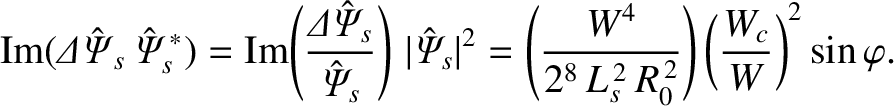(13.10)

Here,is the major radius of the plasma, and use has been made of Equations (5.129) and (13.1). The previous equation can be combined with Equations (3.189)–(3.191) and (13.2) to give the following set of equations that govern the time evolution of the magnetic island chain's helical phase: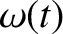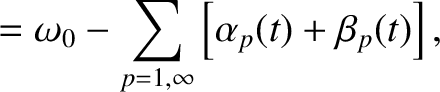(13.11)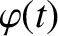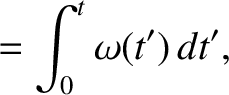(13.12)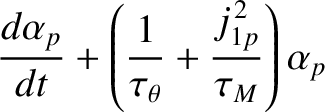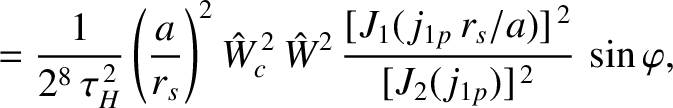(13.13)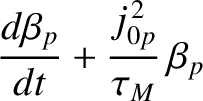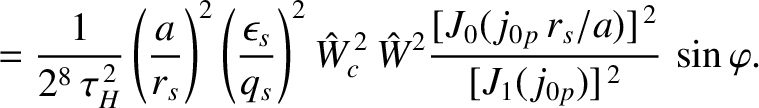(13.14)

Here,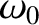is the natural frequency of the magnetic island chain (i.e., the chain's rotation frequency in the absence of the resonant magnetic perturbation),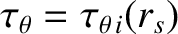the ion neoclassical poloidal flow-damping time at the rational surface [see Equation (2.332)], and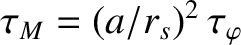. Moreover,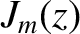is a Bessel function, and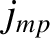denotes its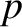th zero . In deriving the previous equations, use has been made of Equations (4.23), (5.43), and (5.129).

Table 13.2: Characteristic parameters governing the time evolution of the width and phase of a magnetic island chain in a low-field and a high-field tokamak fusion reactor.
 Low-Field High-Field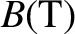5.0 12.0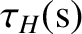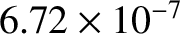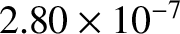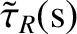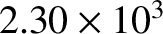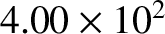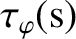1.60 0.278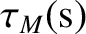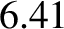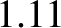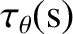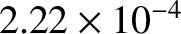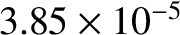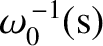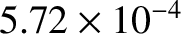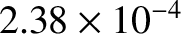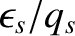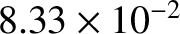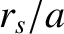0.5 0.5